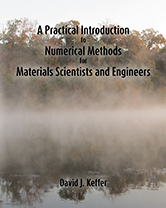A Practical Introduction to Numerical Methods for Materials Scientists and Engineers A Practical Introduction to Numerical Methods for Materials Scientists and Engineers David J. Keffer Professor of Materials Science & Engineering University of Tennessee Knoxville, TennesseeDescription: We live in a world where our time is limited. As materials scientists and engineers, we fulfill the role of problem-solvers in society. Our success in this endeavor depends upon our ability to solve a particular problem within a given amount of time, which in turns depends upon our familiarity with and access to the standard tools of the trade. A rudimentary knowledge of the tools may allow us to provide a crude solution, whereas a more thorough knowledge of the tools may allow us to generate a more elegant and satisfying solution. Today, many of the problems posed to materials scientists and engineers involve a computational component. Thus the analysis of a problem can be broken down into problem formulation and problem solution. Problem formulation requires creativity to construct a framework in which the material issue under investigation can be property understood. Problem formulation is also the most crucial step because through the formulation of the model we dictate the physics present in the model. If an important element is omitted in this step, the solution of the model either will not provide any insight into the problem at hand or worse yet provide erroneous guidance. Problem formulation is also the most exciting part of any investigation because our imagination can run wild at this point, leading us to ingenious approaches that not only solve the problem but help us to establish the reputation for creativity and excellence toward which we strive. However, the formulation of the model must be followed by solution of the model. Today, there are standard tools for solving virtually any variety of mathematical equation, due to the cumulative labors of previous generations of mathematicians and computer scientists. With a working familiarity of these tools, the solution of most models should be simply a matter of methodical routine. While it is true that there is the opportunity to invoke our creativity in the solution of more advanced materials problems requiring specialized numerical techniques, there remains a broad swath of materials problems that can be routinely solved using a relatively small toolbox of standard numerical techniques. There is the opportunity for great empowerment of the student with this approach. What we hope to avoid is a situation where the rigor in the formulation of the model is sacrificed in order to achieve a mathematically simpler expression, which can be solved with a very limited set of tools. What we hope to achieve is a situation where the formulation proceeds without regard for the ease of numerical solution. Once the model is rigorously formulated, the appropriate numerical solution is then identified. Thus the science guides the numerical techniques, rather than the other way around. The philosophy espoused in this book is to equip the student with a compact but broadly applicable set of practical problem-solving tools such that the student emerges at the end of the course with the belief, "If I can formulate the problem as a mathematical model, I can solve it." This book is currently used to cover the second half of a semester-long course in applied statistics and numerical methods targeted at students in their junior year. The first half of the course, which covers applied statistics, uses a matching textbook, A Practical Introduction to Applied Statistics for Materials Scientists and Engineers. This book assumes a knowledge of differential and integral calculus. It also assumes some familiarity with ordinary differential equations. This book covers: numerical differentiation numerical integration solution of algebraic equations systems of linear algebraic equations eigenanalysis linear regression non-linear algebraic equations single nonlinear algebraic equations systems of nonlinear algebraic equations optimization solution of ordinary differential equations single nonlinear ordinary differential equations systems of nonlinear ordinary differential equations initial value problems boundary value problems This book does not cover: solution of partial differential equations solution of integral equations signal filtering, including Fourier transforms countless other more advanced numerical topics length: 149 pages paperback size: 8 in x 10 in paperback publication date: September 21, 2015 paperback ISBN-13: 978-1517356675 paperback ISBN: 1517356679 ebook publication date: N.A. ebook ISBN-13: N.A. poison pie publishing house catalog number: PP-030-T buy it in the shop